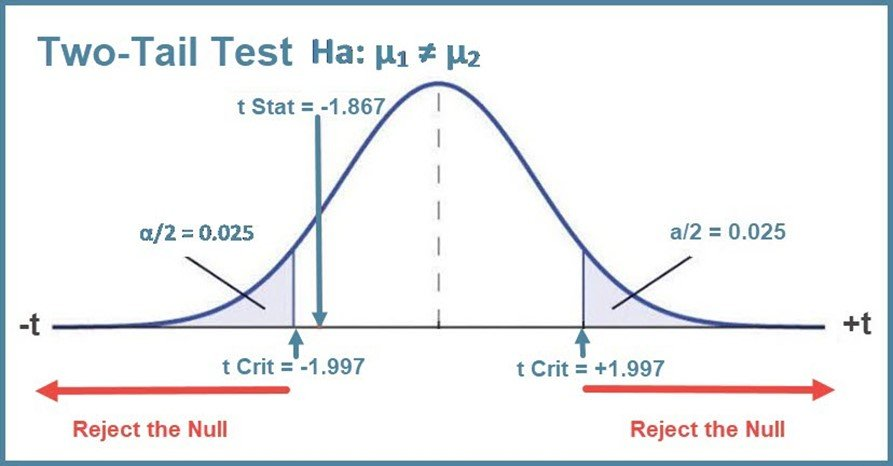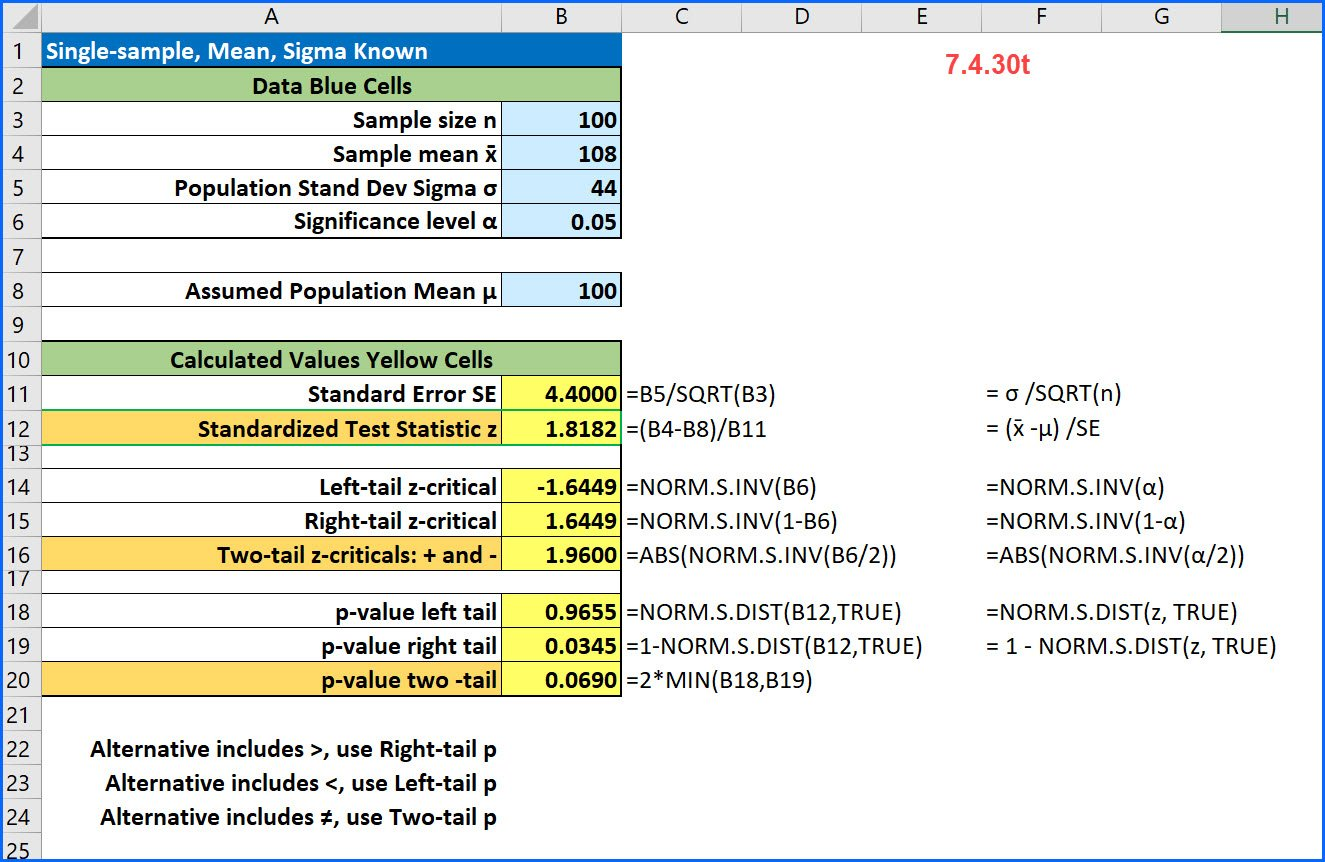# Front

## Empirical Rule and z-score Probability

Empirical Rule The Empirical Rule applies to a normal, bell-shaped curve than is symmetrical about the mean. It states that within one standard deviation of the mean (both left-side and right-side) there is about 68% of the data; within two standard deviations of the mean (both left-side and right-side) there is about 95% of the data; …## Finite Population Correction factor

The Finite Population Correction Factor, sometimes just called the FPC factor, is used when the sample size is large relative to the population size. For most situations, the population is so large, typical sample sizes are far too small to worry about the need for the FPC. The guidance is that we need to use …## Tail of the Test: Interpreting Excel Data Analysis t-test output

Excel’s Data Analysis ToolPak has three tools for running tests of hypotheses using the t-distribution – t-tests. The output from the tools can be a bit confusing because, unlike other statistical software, these do not allow you to specify the “tail of the test” before you run the analysis. Here is how Microsoft explains how …## Single-sample z-test for the mean [7.4.30t]

Here is a common problem from intro stats: [7.4.30t] A random sample of 100 observations from a population with a standard deviation of 44 yielded a sample mean of 108. Test the null hypothesis that μ = 100 against the alternative that μ > 100 at an alpha of 0.05. Here because the alternative contains …## Why is the Standard Error Equal to Sigma Divided by the Square Root of n?

Every time I teach the Central Limit Theorem, I get questions from students on why we divide the population standard deviation, sigma, by the square root of the sample size to calculate the standard deviation of the sampling distribution which we call the standard error. Recall that the equation for the standard error is where …## “Easy” Excel Inverse Triangular Distribution for Monte Carlo Simulations Redux

Back in the dark ages when access to computers was not all that common, I was faced with developing a project schedule for, to me, a complex construction project. I was not that long out of school, so I sought out my boss with the hope he would give me some guidance on how to …